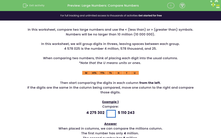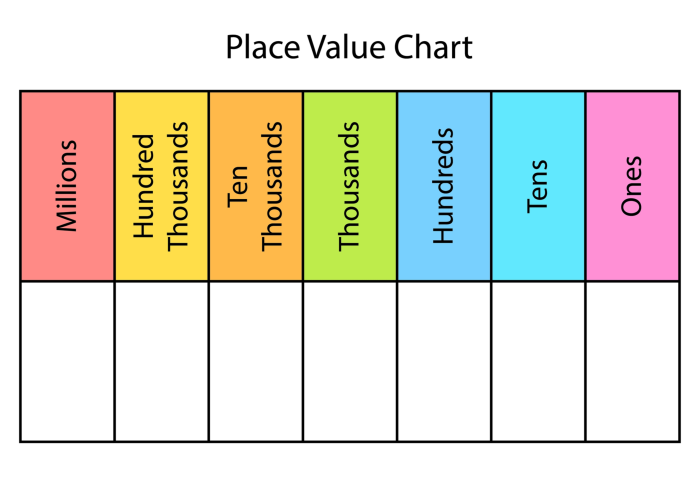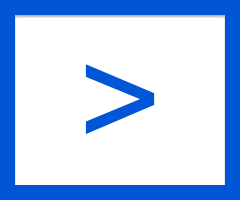# Compare Large Numbers Using Knowledge of Place Value

In this worksheet, students will compare numbers up to 10,000,000, using the < or > sign between the two given numbers.Key stage:  KS 2

Curriculum topic:   Number: Number and Place Value

Curriculum subtopic:   Determine Digit Value to 10 000 000

Popular topics:   Place Value worksheets

Difficulty level:#### Worksheet Overview

In this activity, we will compare two large numbers and use the < (less than) or > (greater than) symbols.

Numbers will be no larger than 10 million (10,000,000).

We will group digits in threes, placing commas between each group.

4,578,025 is the number 4 million, 578 thousand, and 25.

When comparing two numbers, think of placing each digit into the usual columns.Then start comparing the digits in each column from the left.

If the digits are the same in the column being compared, move one column to the right and compare those digits.

Example 1

Compare:

4,275,3025,110,243

When placed in columns, we can compare the millions column.

The first number has only 4 million.

The second number has 5 million.

4,275,3025,110,243

Example 2

Compare:

4,275,3024,210,243

When placed in columns, we can compare the millions column.

They are both 4 million.

So we go to the next column - the hundred thousands column.

They are both 2 hundred thousand.

So we go to the next column – the ten thousands column.

The first number has 7 in this column.

The second number has 1 in this column.

4,275,3024,210,243

Example 3

Compare:

275,3022,710,243

When placed in columns, the number on the right has the digit 2 in the millions column.

The number on the left doesn't have a digit in the millions column. Call it zero.

0 < 2

275,3022,710,243

Right, let's get started!

### What is EdPlace?

We're your National Curriculum aligned online education content provider helping each child succeed in English, maths and science from year 1 to GCSE. With an EdPlace account you’ll be able to track and measure progress, helping each child achieve their best. We build confidence and attainment by personalising each child’s learning at a level that suits them.

Get started